Next: Complexity of Some Up: Parallel Algorithm Analysis Previous: Simple and Regular

## CM-2 Communications Model

The CM-2 contains from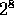to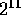Sprint nodes interconnected by a binary hypercube network. Each Sprint node consists of two chips of processors, each with 16 bit-serial processing elements. Thus, the CM-2 has configurations ranging between 8K and 64K, inclusive, bit-serial processors. In this analysis, we view each Sprint node as a single 32-bit processing node.

The CM-2 programming model of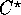uses a canonical data layout similar to that on the CM-5 described in Section 2.2.1\ (, , , ). The only difference on the CM-2 and CM-5 is that the Sprint node, or major grid, portion of each data element address is in reflected binary gray code, insuring that nearest neighbor communications are at most one hop away in the hypercube interconnection network.

We now analyze the complexity for a regular grid shift on the CM-2. Each node holds a contiguous subgrid of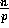data elements. During a grid shift, O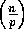elements remain local to each Sprint node, giving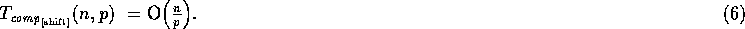Each Sprint node will send border elements to their destination node. By the canonical layout, we are assured that this destination is an adjacent node in the hypercube. Every Sprint node will send and receive elements along unique paths of the network. In this model, we neglect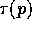since we are using direct hardware links which do not incur the overhead associated with packet routing analyses. Thus, each Sprint node will need to send and receive O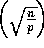elements, yielding a communications complexity of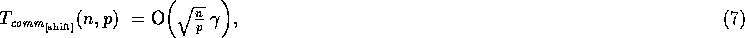where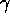is the CM-2 transmission rate into the network.

Therefore, on a CM-2 with p Sprint nodes, a regular grid shift of a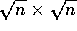data array has the following time complexity analyses:As shown, a regular grid shift on the CM-2 is scalable for the array size and the machine size.Next: Complexity of Some Up: Parallel Algorithm Analysis Previous: Simple and Regular